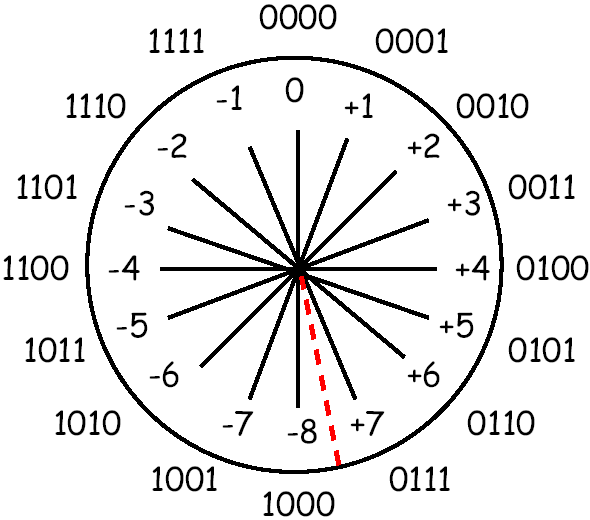Arithmetic Operations on Binary Numbers

Because of its widespread use, we will concentrate on addition and subtraction for Two's Complement representation.

The nice feature with Two's Complement is that addition and subtraction of Two's complement numbers works without having to separate the sign bits (the sign of the operands and results is effectively built-into the addition/subtraction calculation).

Remember: −2n−1 ≤ Two's Complement ≤ 2n−1 − 1

−8 ≤ x ≤ +7

−128 ≤ x ≤ +127

−32768 ≤ x ≤ +32767

−2147483648 ≤ x ≤ +2147483647

What if the result overflows the representation?

If the result of an arithmetic operation is to too large (positive or negative) to fit into the resultant bit-group, then arithmetic overflow occurs. It is normally left to the programmer to decide how to deal with this situation.

Examples: using 8-bit two’s complement numbers.

``` (+3)   0000 0011
+(−8)   1111 1000
-----------------
(−5)   1111 1011
```

``` (−2)   1111 1110
+(−5)   1111 1011
-----------------
(−7) 1 1111 1001 : discard carry-out
```

If 2 Two's Complement numbers are added, and they both have the same sign (both positive or both negative), then overflow occurs if and only if the result has the opposite sign. Overflow never occurs when adding operands with different signs.

 i.e. Adding two positive numbers must give a positive result Adding two negative numbers must give a negative result

Overflow occurs if

• (+A) + (+B) = −C
• (−A) + (−B) = +C

Example: Using 4-bit Two's Complement numbers (−8 ≤ x ≤ +7)

``` (−7)   1001
+(−6)   1010
------------
(−13) 1 0011 = 3 : Overflow (largest −ve number is −8)
```

A couple of definitions:

 Subtrahend: what is being subtracted Minuhend: what it is being subtracted from

Example: 612 - 485 = 127

485 is the subtrahend, 612 is the minuhend, 127 is the result

Two's Complement Subtraction

Normally accomplished by negating the subtrahend and adding it to the minuhend. Any carry-out is discarded.

Example: Using 8-bit Two's Complement Numbers (−128 ≤ x ≤ +127)

``` (+8) 0000 1000               0000 1000
−(+5) 0000 0101 -> Negate -> +1111 1011
-----                       -----------
(+3)                       1 0000 0011 : discard carry-out
```

Overflow Rule for Subtraction

If 2 Two's Complement numbers are subtracted, and their signs are different, then overflow occurs if and only if the result has the same sign as the subtrahend.

Overflow occurs if

• (+A) − (−B) = −C
• (−A) − (+B) = +C

Example: Using 4-bit Two's Complement numbers (−8 ≤ x ≤ +7)

Subtract −6 from +7

```     (+7) 0111               0111
−(−6) 1010 -> Negate -> +0110
----------              -----
13                     1101 = −8 + 5 = −3 : Overflow
```

Number Circle for 4-bit Two's Complement numbers

Numbers can be added or subtracted by moving round the number circle

• Anti-Clockwise for subtraction (addtion of a negative number)Overflow occurs when a transition is made

• from +2n−1−1 to −2n−1 when adding
• from −2n−1 to +2n−1−1 when subtracting

Two's Complement Summary

Subtraction

Overflow

• Occurs when adding two positive numbers produces a negative result, or when adding two negative numbers produces a positive result. Adding operands of unlike signs never produces an overflow
• Notice that discarding the carry out of the most significant bit during Two's Complement addition is a normal occurrence, and does not by itself indicate overflow
• As an example of overflow, consider adding (80 + 80 = 160)10, which produces a result of −9610 in 8-bit two's complement:

```  01010000 =  80
+ 01010000 =  80
--------------
10100000 = −96 (not 160 because the sign bit is 1.)

(largest +ve number in 8 bits is 127)
```

Multiplication and Division

No problem with unsigned (always positive) numbers, just use the same standard techiques as in base 10 (remembering that x[n] × y[n] = z[2n])

• Multiplication Example 11001012 × 1111012 (10110 × 6110)

```      1100101   10110
× 111101  × 6110
-------
1100101
+1100101
+1100101
+1100101
+1100101
-------------
?????????????
-------------
```

Easier to use intermediary results:

```      1100101   10110
× 111101  × 6110
-------
1100101
+1100101
----------
111111001
+1100101
------------
10100100001
+1100101
-------------
101101110001
+1100101
-------------
1100000010001 = 409610 + 204810 + 1610 + 1 = 616110
-------------```
• Division Example: 1001012 ÷ 1012 (3710 ÷ 510)

```       111 result = 710
-------
101)100101
−101
---
1000
−101
---
111
−101
---
10 remainder = 210
---
```

Multiplication in Two's complement cannot be accomplished with the standard technique since, as far as the machine itself is concerned, for Y[n]:

−Y  º  0 − Y  º  2n − Y

since, when subtracting from zero, need to "borrow" from next column leftwards.

Example:

 −1910 in 8-bits = (28 − 19)10 = 25610 − 1910 = 23710 = 111011012 unsigned = −27 + 26 + 25 + 23 + 22 + 20 = −128 + 64 + 32 + 8 + 4 + 1 = −128 + 109 = −1910 in Two's Complement

Consider X × (−Y)

Internal manipulation of −Y is as 2n − Y

Therefore X × (−Y)  =  X × (2n − Y)  =  2n × X − X × Y

However the expected result should be 22n − (X × Y)

since x[n] × y[n] = z[2n]

Can perform multiplication by converting the Two's Complement numbers to their absolute values and then negating the result if the signs of the operands are different.

Similar for Two's Complement division. Can convert the operands to their absolute values, perform the division, and then negating the result if the signs of the operands are different.

Most contemporary architectures implement more sophisiticated algorithms for multiplication and division of Two's Complement numbers.

Beyond the scope of this course!

[ Index ]

last updated: 21-Oct-02 Ian Harries <ih@doc.ic.ac.uk>